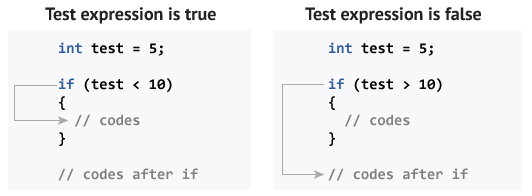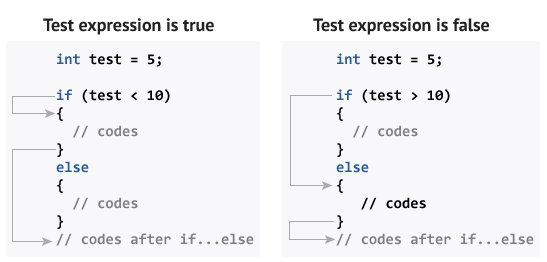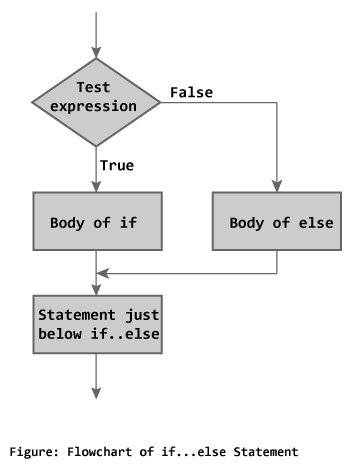C++ if, if...else and Nested if...else

In this article, you will learn to create decision making statements in a C++ program using different forms of if..else statement.Contents
if Statement
if...else Statement
Nested if...else Statement
Conditional Operator

C++ if Statement

if (testExpression)
{
// statements
}

The if statement evaluates the test expression inside parenthesis.

If test expression is evaluated to true, statements inside the body of if is executed.

If test expression is evaluated to false, statements inside the body of if is skipped.

How if statement works?Flowchart of if StatementAbove figure describes the working of an if statement.

Example 1: C++ if Statement

// Program to print positive number entered by the user
// If user enters negative number, it is skipped

#include <iostream>
using namespace std;

int main()
{
int number;
cout << "Enter an integer: ";
cin >> number;

// checks if the number is positive
if ( number > 0)
{
cout << "You entered a positive integer: " << number << endl;
}

cout << "This statement is always executed.";
return 0;

}

Output 1

Enter an integer: 5
You entered a positive number: 5
This statement is always executed.

Output 2

Enter a number: -5
This statement is always executed.

C++ if...else

The if else executes the codes inside the body of if statement if the test expression is true and skips the codes inside the body of else.

If the test expression is false, it executes the codes inside the body of else statement and skips the codes inside the body of if.

How if...else statement works?Flowchart of if...elseExample 2: C++ if...else Statement

// Program to check whether an integer is positive or negative
// This program considers 0 as positive number

#include <iostream>
using namespace std;

int main()
{
int number;
cout << "Enter an integer: ";
cin >> number;

if ( number >= 0)
{
cout << "You entered a positive integer: " << number << endl;
}

else
{
cout << "You entered a negative integer: " << number << endl;
}

cout << "This line is always printed.";
return 0;
}

Output

Enter an integer: -4
You entered a negative integer: -4.
This line is always printed.

C++ Nested if...else

The if...else statement executes two different codes depending upon whether the test expression is true or false. Sometimes, a choice has to be made from more than 2 possibilities.

The nested if...else statement allows you to check for multiple test expressions and execute different codes for more than two conditions.

Syntax of Nested if...else

if (testExpression1)
{
// statements to be executed if testExpression1 is true
}
else if(testExpression2)
{
// statements to be executed if testExpression1 is false and testExpression2 is true
}
else if (testExpression 3)
{
// statements to be executed if testExpression1 and testExpression2 is false and testExpression3 is true
}
.
.
else
{
// statements to be executed if all test expressions are false
}

Example 3: C++ Nested if...else

// Program to check whether an integer is positive, negative or zero

#include <iostream>
using namespace std;

int main()
{
int number;
cout << "Enter an integer: ";
cin >> number;

if ( number > 0)
{
cout << "You entered a positive integer: " << number << endl;
}
else if (number < 0)
{
cout<<"You entered a negative integer: " << number << endl;
}
else
{
cout << "You entered 0." << endl;
}

cout << "This line is always printed.";
return 0;
}

Output

Enter an integer: 0
You entered 0.
This line is always printed.

Conditional/Ternary Operator ?:

A ternary operator operates on 3 operands which can be used instead of a if...else statement.

Consider this code:

if ( a < b ) {
a = b;
}
else {
a = -b;
}

You can replace the above code with:

a = (a < b) ? b : -b;

The ternary operator is more readable than a if...else statement for short conditions.